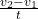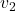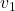## What is the impulse of a 3 kg object that starts from rest and moves to 20 m/s?

Question

What is the impulse of a 3 kg object that starts from rest and moves to 20 m/s?

in progress 0
4 months 2021-07-14T03:53:04+00:00 1 Answers 2 views 0

The impulse on the object is 60Ns.

Explanation:

Impulse is defined as the product of the force applied on an object and the time at which it acts. It is also the change in the momentum of a body.

F = m a

F = m()

⇒     Ft = m()

where: F is the dorce on the object, t is the time at which it acts, m is the mass of the object,is its initialvelocity andis the final velocity of the object.

Therefore,

impulse = Ft = m()

From the question, m = 3kg,= 0m/s and= 20m/s.

So that,

Impulse = 3 (20 – 0)

= 3(20)

= 60Ns

The impulse on the object is 60Ns.